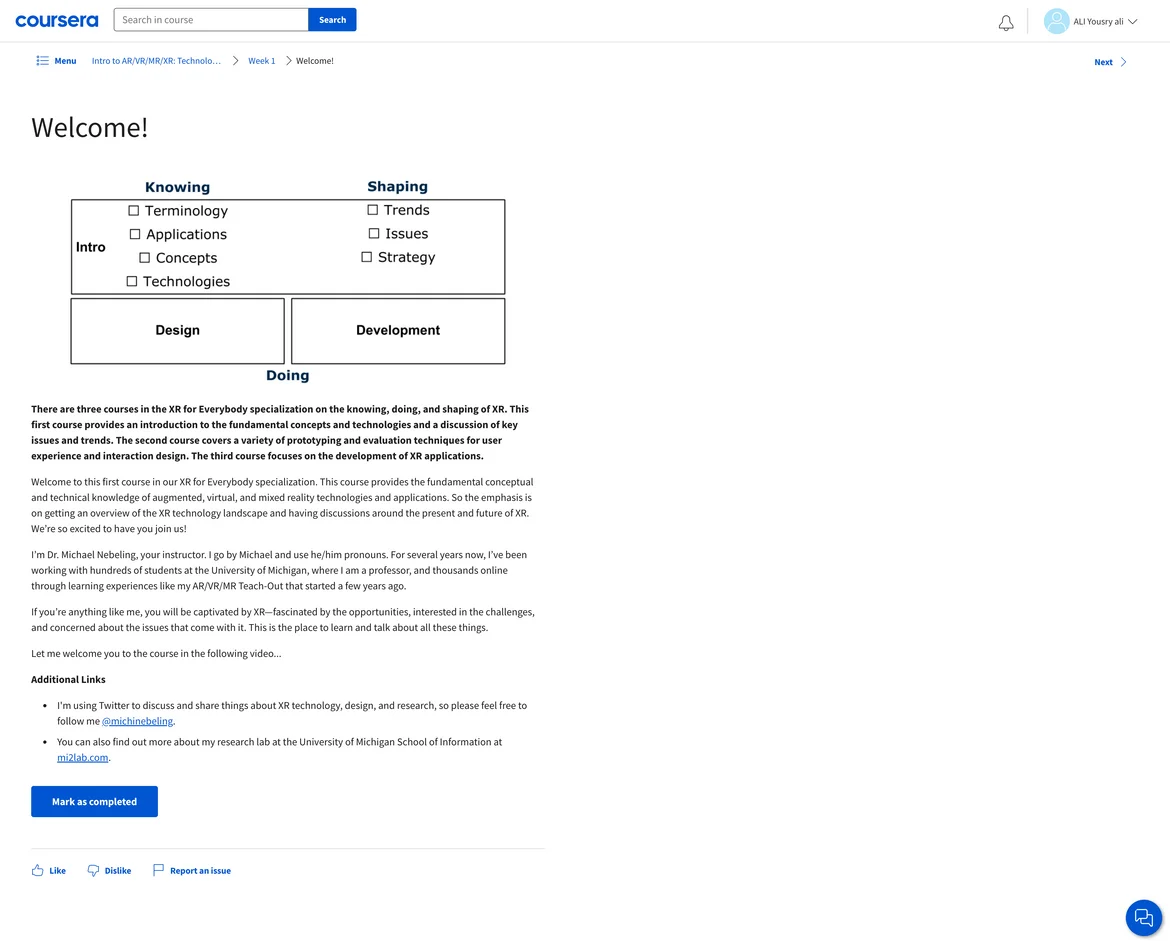29 May 2023

Posted on:

28 May 2023

0

# else statement not working

Can anybody let me know what I put wrong in the following code? becuase although the number is negative the else statement does not work, and the output is your number is positive.

v <- -4
if(v > 0) {
} else {
v <- v*-1
print(v)
} else if(v == 0){
}

Posted on:

29 May 2023

0Instructor
Posted on:

29 May 2023

0

Hi Saeed,

thanks for reaching out! The goal of the code in this case is not to check whether a number is positive or negative, but rather prevent you from entering a negative number. So, the code first checks if your number is >= 0. If yes, it either tells you that it is positive, or that it is exactly 0. Otherwise, if the number is negative, like in your example, it transforms the number  and states that "your number is now positive". The first else statement changes the negative to a positive number with the following line:

`` v <- v*-1``

So, if your output is "your number is now positive" than it is correct. Let me know if you have any further questions.

Best,

365 Eli